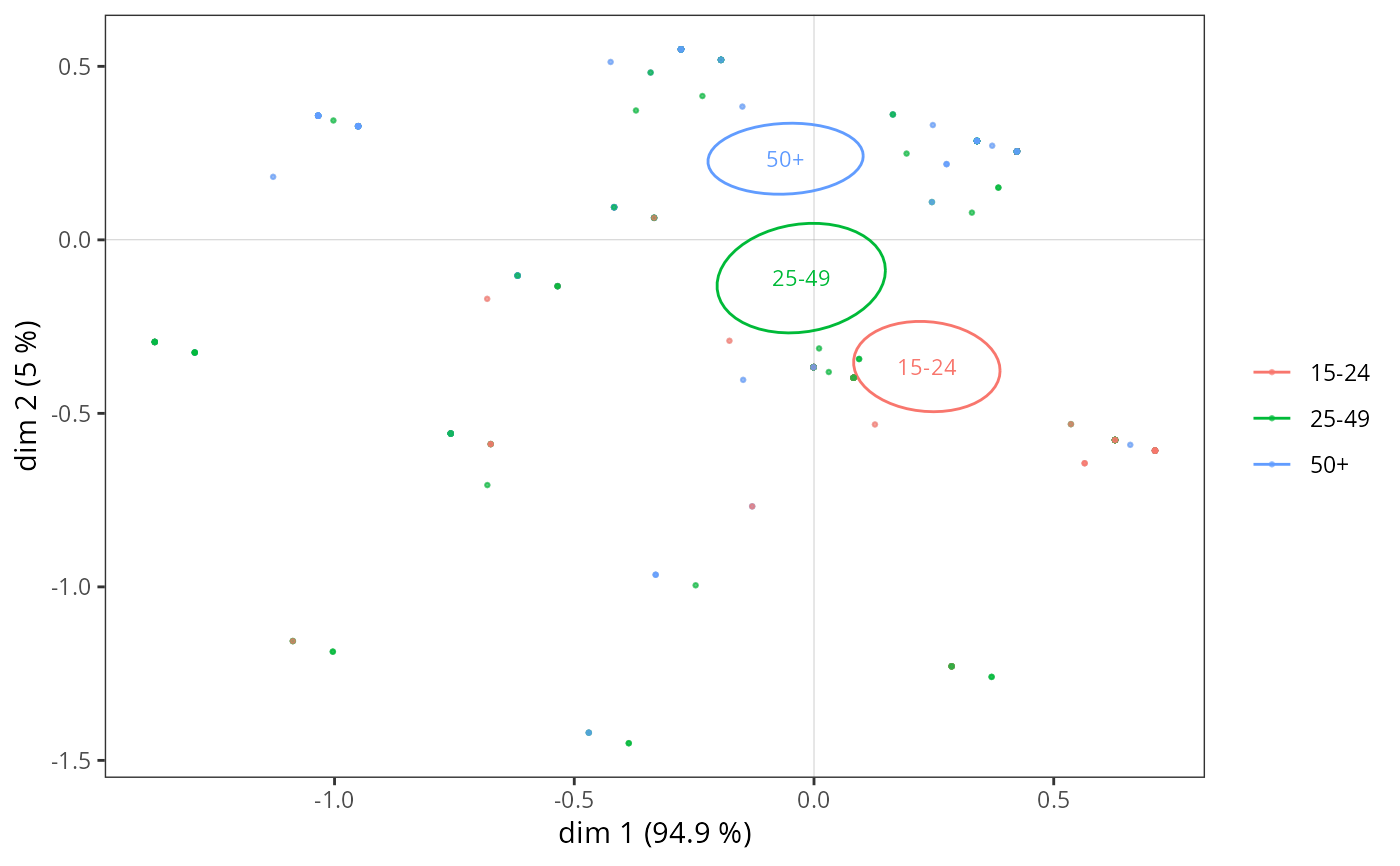Adds confidence ellipses for a categorical variable to a MCA cloud of individuals

ggadd_ellipses(p, resmca, var, sel = 1:nlevels(var), axes = c(1,2),
level = 0.05, label = TRUE, label.size = 3, size = 0.5, points = TRUE,
legend = "right")

## Arguments

p

ggplot2 object with the cloud of individuals

resmca

object of class MCA, speMCA, csMCA, stMCA or multiMCA

var

Factor. The categorical variable used to plot ellipses.

sel

numeric vector of indexes of the categories to plot (by default, ellipses are plotted for every categories)

axes

numeric vector of length 2, specifying the components (axes) to plot. Default is c(1,2).

level

The level at which to draw an ellipse (see stat_ellipse). Default is 0.05, which means 95 percents confidence ellipses are plotted.

label

Logical. Should the labels of the categories be plotted at the center of ellipses ? Default is TRUE.

label.size

Size of the labels of the categories at the center of ellipses. Default is 3.

size

Size of the lines of the ellipses. Default is 0.5.

points

If TRUE (default), the points are coloured according to their subcloud.

legend

the position of legends ("none", "left", "right", "bottom", "top", or two-element numeric vector). Default is right.

## Value

a ggplot2 object

## Details

A confidence ellipse aims at measuring how the "true" mean point of a category differs from its observed mean point. This is achieved by constructing a confidence zone around the observed mean point. If we choose a conventional level alpha (e.g. 0.05), a (1 - alpha) (e.g. 95 percents) confidence zone is defined as the set of possible mean points that are not significantly different from the observed mean point.

## Note

Ellipses are colored according to the categories of the variable, using the default ggplot2 palette. The palette can be customized using any scale_color_* function, such as scale_color_brewer(), scale_color_grey() or scale_color_manual().

## Author

Nicolas Robette

ggcloud_indiv, ggadd_supvar, ggadd_supvars, ggadd_kellipses, ggadd_density, ggadd_interaction, ggsmoothed_supvar, ggadd_chulls, ggadd_corr

## Examples

# specific MCA of Music example data set
data(Music)
junk <- c("FrenchPop.NA", "Rap.NA", "Rock.NA", "Jazz.NA", "Classical.NA")
mca <- speMCA(Music[,1:5], excl = junk)
# confidence ellipses for Age
p <- ggcloud_indiv(mca, col = "lightgrey")